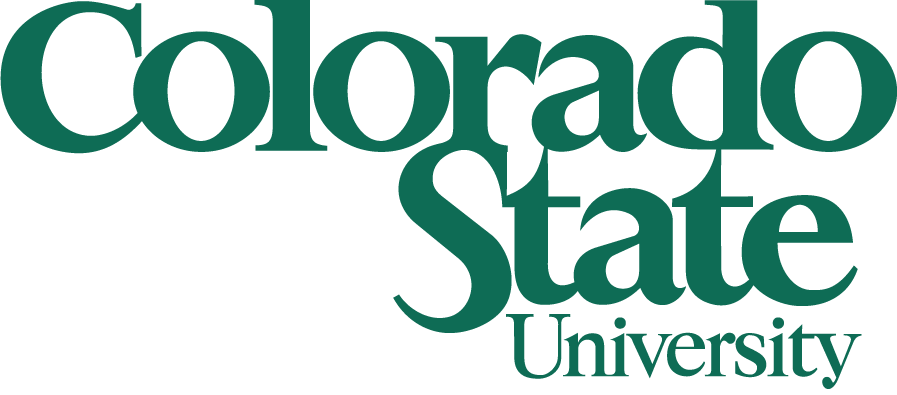# Seminar## Rocky Mountain Algebraic Combinatorics Seminar

### Almost orthogonal vectors

William J Martin
Worcester Polytechnic Institute

How many lines can one arrange through the origin in ten-dimensional space so that the angle between any two is at least 89°? Of course one can do no better than ten and slightly perturbed coordinate axes provide the obvious example. In \mathbbR57, we again can fit only 57 lines, but in \mathbbR58 it is possible to fit 59 lines through the origin in such a way that the angle between any two exceeds 89°. It might then be surprising to learn that the maximum number of lines through the origin in \mathbbRd forming pairwise angles within this one-degree tolerance of 90° grows exponentially as a function of d.

Our problem is to consider functions ε = ε(d) (tending toward zero) and to estimate the largest size F(d,ε) of a set X of unit vectors in \mathbbRd whose pairwise dot products all lie within the interval [−ε,ε]. As indicated above, for ε a positive constant, F(d,ε) is exponential in d. For ε(d) = c/d where c is a positive constant, F(d,ε) is roughly equal to d for large d. So where does exponential growth begin? How about polynomially many lines? Examples will be taken from quantum information theory, compressive sensing, association schemes and coding theory. Tools employed include the linear programming bound of Delsarte, Goethals and Seidel and the probabilistic method.

### Constructions of Q-polynomial Association Schemes

Jason Williford
University of Wyoming

An association scheme is called Q-polynomial if, after suitably reordering the idempotents, the idempotent Ei is a degree i polynomial of E1, where multiplication is done using the Schur product. The notion of Q-polynomial schemes is formally dual to schemes generated by distance-regular graphs. However, the theory of Q-polynomial schemes is much less understood than its dual counterpart. In this talk, we will discuss the known Q-polynomial schemes, including a previously unknown family.

Weber 223
4–6 pm
Friday, April 25, 2014
(Refreshments in Weber 117, 3:30–4 pm)

This is a joint Denver U / UC Boulder / UC Denver / U of Wyoming / CSU seminar that meets biweekly. Anyone interested is welcome to join us at a local restaurant for dinner after the talks.

### Previous Seminars:

April 11, 2014
Victor Pambuccian, George Shakan
March 7, 2014
Nathan Lindzey, Jens Harlander
February 21, 2014
Ross McConnell, Anton Betten
November 22, 2013
Justin Hughes, Josh MaglioneDepartment of Mathematics